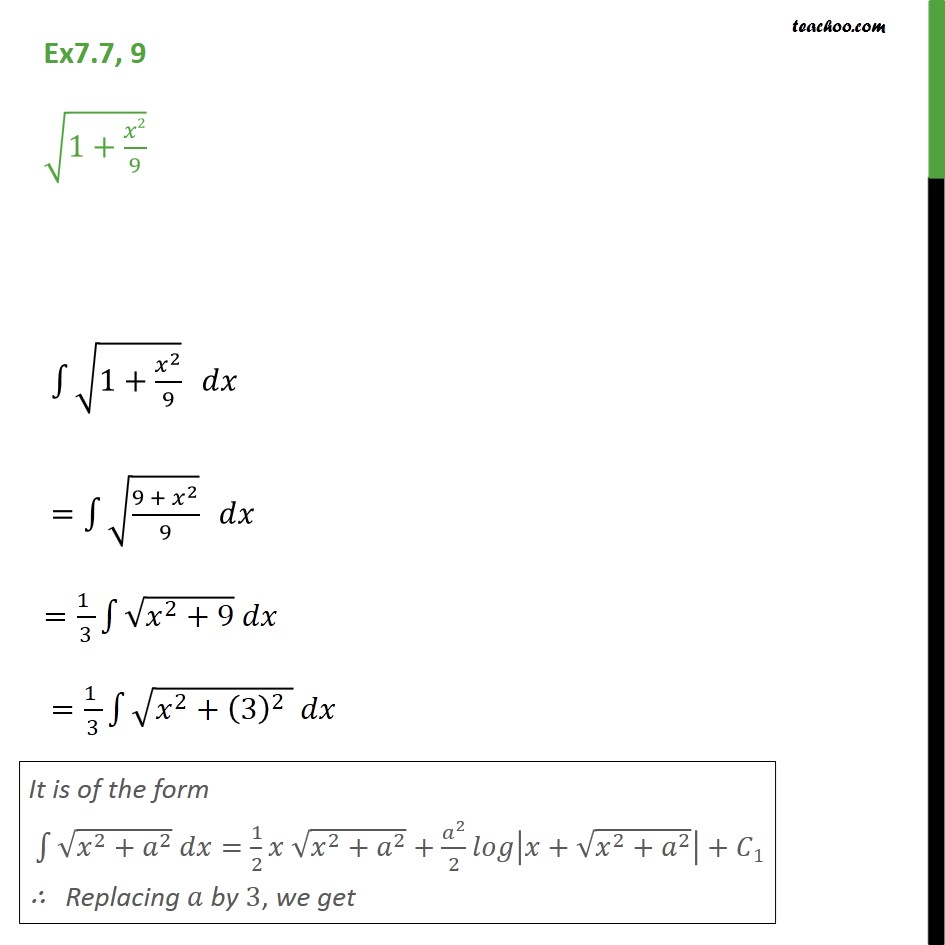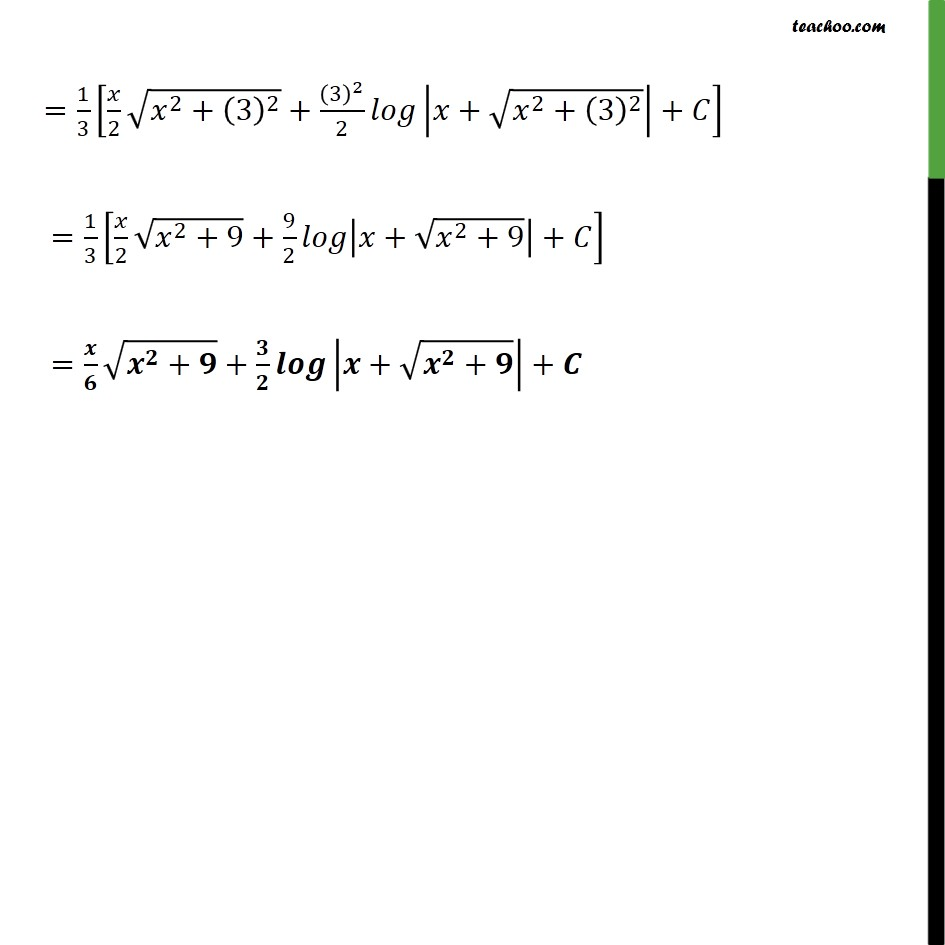Ex 7.7

Chapter 7 Class 12 Integrals
Serial order wiseLearn in your speed, with individual attention - Teachoo Maths 1-on-1 Class

### Transcript

Ex7.7, 9 1+ 2 9 1+ 2 9 = 9 + 2 9 = 1 3 2 +9 = 1 3 2 + 3 2 = 1 3 2 2 + 3 2 + 3 2 2 + 2 + 3 2 + = 1 3 2 2 +9 + 9 2 + 2 +9 + = + + + + +